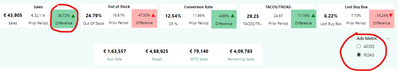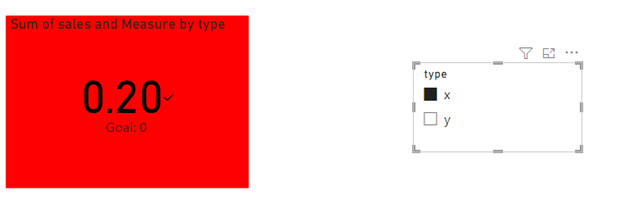cancel
Showing results for
Did you mean:Regular Visitor

## Two rules for a KPI

Hello beautiful people,
I have a scenario, not sure how to proceed further.

I have a KPI which changes its color depending upon the value (Green if positive and red for negative). Now I have a toggle, X and Y and the KPI has to behave differently (changing its color) when switch these toggles.
For Example, Sales KPI should be in Green color when I chose Y filter measure and the same KPI should be in Red when I select X filter measure, basically having two rules for the same KPI. Any leads how do I go about this ?1 ACCEPTED SOLUTIONCommunity Support

``````color = SWITCH(TRUE(),
MAX('Table'[sales])>0 && SELECTEDVALUE('Table'[type])="x","green",
MAX('Table'[sales])<0 && SELECTEDVALUE('Table'[type])="x","red",
MAX('Table'[sales])>0 && SELECTEDVALUE('Table'[type])="y","red",
MAX('Table'[sales])<0 && SELECTEDVALUE('Table'[type])="y","green",
"white")``````

Best Regards,

Neeko Tang

If this post  helps, then please consider Accept it as the solution  to help the other members find it more quickly.

4 REPLIES 4Frequent Visitor

Thanks Niko for your quick response 🙂
I should've been more clear about the problem.
So there needs to be two rules for the KPI. In your example,
1. X : Cell should be green if sales is positive and red if negative
2. Y : Cell should be red if sales is positive and green if negative.Community Support

``````color = SWITCH(TRUE(),
MAX('Table'[sales])>0 && SELECTEDVALUE('Table'[type])="x","green",
MAX('Table'[sales])<0 && SELECTEDVALUE('Table'[type])="x","red",
MAX('Table'[sales])>0 && SELECTEDVALUE('Table'[type])="y","red",
MAX('Table'[sales])<0 && SELECTEDVALUE('Table'[type])="y","green",
"white")``````

Best Regards,

Neeko Tang

If this post  helps, then please consider Accept it as the solution  to help the other members find it more quickly.Frequent Visitor

Thanks Neeko, this solution worked. Thank you so much 🙂Community Support

According to your description, here are my steps you can follow as a solution.

(1) This is my test data.(2) We can create a measure.

``color = IF(MAX('Table'[sales])>0 && SELECTEDVALUE('Table'[type])="y","green","red")``

(3)Set the conditional formatting as shown in the following figure.(3) Then the result is as follows.Best Regards,

Neeko Tang

If this post  helps, then please consider Accept it as the solution  to help the other members find it more quickly.